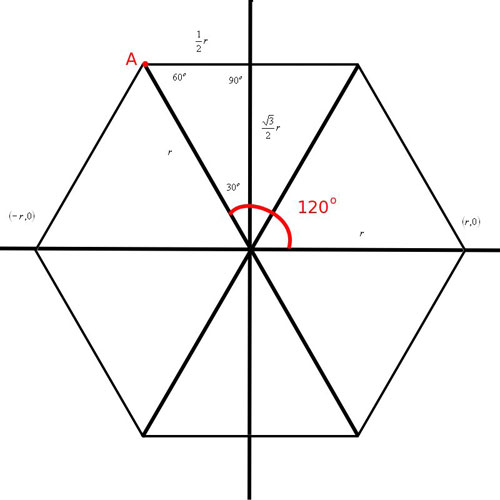SEARCH HOMEMath Central Quandaries & QueriesQuestion from Martin, a teacher: Given a regular hexagon with center on the origin of an x-y plane with coordinate (r,0) on the x axis, we are to express the coordinates of "A" located at 120 deg from (r,0) in terms of "r".Hi Martin,
It is easiest to find the coordinates of A if you think of the hexagon as 6 equilateral triangles. Each side of these triangles are of length r. Since the y axis creates a 30-60-90 triangle where A is one of the vertices, it is pretty easy to find the remaining sides of the triangle. The length of these sides will help you find the coordinate point for A.JaniceMath Central is supported by the University of Regina and The Pacific Institute for the Mathematical Sciences.